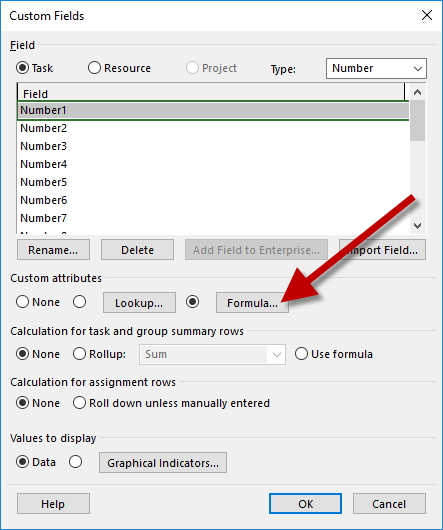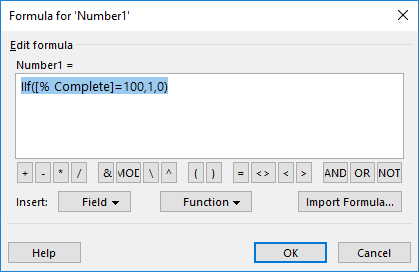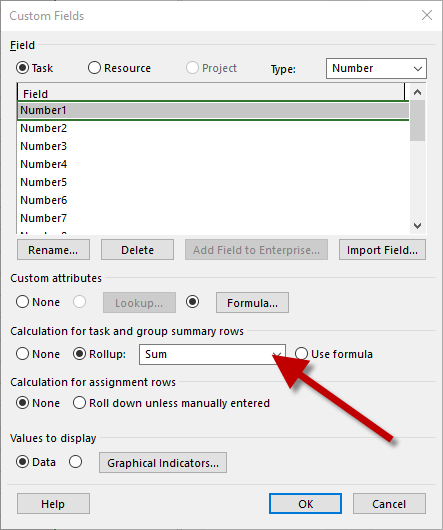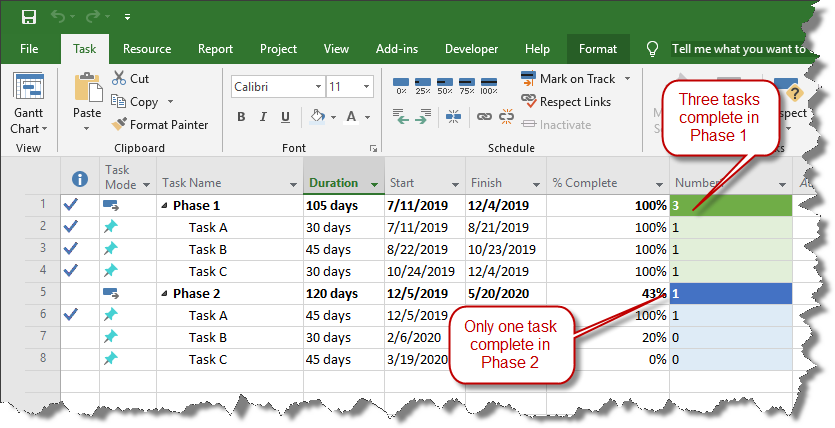[email protected] +1.303.779.0344

# Count Completed Activities in Microsoft Project

## How to get a total count of all of the completed tasks in your Microsoft Project plan.

When you mark a task as complete in Microsoft Project, the summary task above it usually shows a percentage of overall completion. But what if you want a simple count of how many activities have been completed and how many have not?

1. Start with a simple Microsoft Project plan. This project has two phases. The % Complete field indicates which individual tasks are complete and which are not:2. Instead of tracking completion as percentage, we want to count a task when it's complete and to skip it when it's not. To do that, we'll add a custom number column:Click on Add New Column then scroll down to Number1.

3. Initially, the custom number field will contain all zeros. To update the number column based on whether or not the task is complete, we want to write a simple formula. Right-click on the header for the Number1 field and choose Custom Fields:4. On the Custom Fields Screen, click the Formula button:5. We'll write a simple Microsoft Project formula that will set the value of Number1 to 1 when the percent complete value is 100%. For anything less than 100%, Number1 will be zero.Here is the formula in plain text:

IIf([% Complete]=100,1,0)

6. In its current state, each line in the project schedule will get either a one or a zero based on its status. However, we want to sum all of those values up, so for summary tasks, instead of looking at the percentage of the summary task itself, we want to add up the count of all the completed tasks underneath it.

To do that, instead of letting the same formula apply to the summary task, we want to have summary tasks do a summary of the formula for their children. Locate the Calculation for task and group summary rows setting and change it to Rollup with a Sum calculation in the dropdown:7. Click OK and the formula will do its work:The first phase of the project, colored in green, has three completed tasks, so the total count next to the Phase 1 summary task is correctly noted as three.

The second phase of the project, colored in blue, has only one completed task. The second task is at 20% and the third task hasn't started, so the total count next to the Phase 2 summary task is one.

8. If you want to see the overall completed task count for the entire project, not just each phase, then you need to turn on the project summary task. To do this, go to the Format tab and turn on the Project Summary Task box:The project summary task, in orange, counts a grand total of four completed tasks in the project plan. Notice that only actual tasks are counted here; the phase-level summary tasks are not included in the overall task completion count, since they are not really tasks in their own right.